Welcome to Doom9's Forum, THE in-place to be for everyone interested in DVD conversion. Before you start posting please read the forum rules. By posting to this forum you agree to abide by the rules.Doom9's ForumRegion-based structure line detection for cartoons (Cartoons mask)
 Register FAQ Calendar Search Today's Posts Mark Forums Read14th December 2021, 04:45 #1  |  Link Blankmedia Registered User   Join Date: Oct 2011 Location: Dans le nord Posts: 62 Region-based structure line detection for cartoons (Cartoons mask) Does anyone knows if there's an implementation of this mask in avisynth or if it's possible : https://link.springer.com/content/pd...015-0007-3.pdf I'm looking for a good cartoon mask, and I don't ever seem to find exacly what I'm looking for. Classic edge detection will create two "line mask" for every thick cartoon line, which is logical. I've tried mt_edge("cartoon") with different threshold and cleaned them with mt_hysteresis like in Hystoria, but there's always something. Like the eyes or black hair of a character will only get edge detected upon a certain distance so the interior of the eyes or hair will not get masked. Then if you apply a dehalo in can create a weird gradiant in the black.14th December 2021, 15:53 #2  |  Link SaurusX Registered User   Join Date: Feb 2017 Posts: 113 One option is to combine the edge mask with a luma mask set to detect the darkest parts of the image, the animation lines. The dark parts of the image are generally those that need the least filtering in any case, so excluding them is only rarely a problem.14th December 2021, 16:18 #3  |  Link VoodooFX Banana User   Join Date: Sep 2008 Posts: 757 Examples in that paper looks nice, maybe someone can implement a plugin based on this paper. __________________ InpaintDelogo, Darkmode User, JerkyWEB Fixer Last edited by VoodooFX; 14th December 2021 at 17:18.15th December 2021, 05:01   #4  |  Link
Blankmedia
Registered User

Join Date: Oct 2011
Location: Dans le nord
Posts: 62
Quote:
 Originally Posted by SaurusXOne option is to combine the edge mask with a luma mask set to detect the darkest parts of the image, the animation lines. The dark parts of the image are generally those that need the least filtering in any case, so excluding them is only rarely a problem.

Thanks for this, I tougth about something like that but didn't know how to phrase it. Your comment made me narrow my search and I stumbled upon lumamask by dogway. It helped a lot.

I'm not familiar with avisynth expressions so I can't really fine tune mask by myself. One time I managed to create a not so bad mask. But it was really ressource intensive.

Code:
```clip_source = last
clip_s = clip_source
clip_Y =clip_source.converttoy().minideen().vsTTempSmooth()
clip_T = clip_s.TEMmod(threshY=2, threshC=20, type=1, link=2, chroma=1, preblur=True).Converttoy(matrix="Rec709").RemoveGrain(mode=11, planar=false).mt_inpand_multi(mode="ellipse",sw=1,sh=1).aWarpSharp2(Blur=4,Type=1,Depth=9,Chroma=2).mt_deflate()
#~ .mt_expand_multi(mode="ellipse",sw=2,sh=2).mt_inpand_multi(mode="ellipse",sw=4,sh=4).aWarpSharp2(Blur=4,Type=1,Depth=9,Chroma=2).mt_inflate().
a = mt_edge(clip_Y,"cartoon",thY1=1.5,thy2=1.75).Removegrain(27)
b = mt_edge(clip_Y, "cartoon",thY1=1.1,thy2=30).Removegrain(27)
c=mt_hysteresis(a, b).Removegrain(27)
#~ mt_hysteresis(c, clip_t).Removegrain(27)
d = mt_hysteresis(clip_T, c).Removegrain(27)#.mt_inpand_multi(mode="ellipse",sw=0,sh=0)
e = mt_hysteresis(d, clip_T).mt_inpand_multi(mode="ellipse",sw=1,sh=1)
masque = mt_hysteresis(e,c).mt_deflate()
masque = merge(masque.Blur(1), clip_T).mt_inpand_multi(mode="ellipse",sw=1,sh=1)

denoised_clip = clip_source.MCTemporalDenoise(settings="very low", radius=2, Chroma=False, edgeclean=true, ecrad=6, stabilize=true, maxr=2, AA=True, useEEDI2=True, maxd =24, protect=True)

clip_dark =  clip_s.Blur(1).Blur(1).drk(100).LSFmod(defaults="slow", strength=300,soft=-2,  ss_x=1.5, edgemode=1, preblur="FFT3Dfilter(sigma=4,plane=0)")
denoised = mt_merge(denoised_clip,clip_dark , masque)```
I'm shooting blind and hope to hit.15th December 2021, 07:53   #5  |  Link
Blankmedia
Registered User

Join Date: Oct 2011
Location: Dans le nord
Posts: 62
Quote:
 Originally Posted by VoodooFXExamples in that paper looks nice, maybe someone can implement a plugin based on this paper.
Yeah, me too!18th December 2021, 07:29 #6  |  Link Blankmedia Registered User   Join Date: Oct 2011 Location: Dans le nord Posts: 62 I tried to create good reliable edgemask for multiple sources (Simpsons dvd and magic school bus old rip. Y played, with lumask, fltmask, mt_edge (cartoon, Robert, min/max.) Tcanny, Vstedsge Still not* sure I'm understanding all . I also tried to create a polynomial function (expression and mtlut) that would keep high luma, and would pull down black pixel so they would be easier to detect, but it didn't work. I think I'm gonna need help. This is the source file, if it's detecting the edge of the bus on frame 45 it would be nice. https://mega.nz/file/R1pwHLRS#hU-kS8...UeeF-PeFICo6dI Edited: for clarity, missing word etc. Last edited by Blankmedia; 18th December 2021 at 16:48.20th December 2021, 06:16 #7  |  Link Blankmedia Registered User   Join Date: Oct 2011 Location: Dans le nord Posts: 62 Am I not getting responses : A) because my question is unclear; B) there's still good information somewhere on the web that you guys think I should have been able to figure it out; C) I might be literally slow brained these past week and not taking the problem correctly. I know you are nice people, I see it in threads. It's been a while since I've been here so I lost touch with the community. So I assume I'm the one in the wrong here . I was out for a pack of smokes, took me couple years to get back kids. Thanks to you all. I leave you a sweet spiritual prayer. May your Ram be big, may your cpu be fast, may the cost storage fall down. Merry Christmas Envoyé de mon Pixel 6 en utilisant Tapatalk20th December 2021, 15:29 #8  |  Link anton_foy Registered User   Join Date: Dec 2005 Location: Sweden Posts: 497 Well to simplify it and I may be wrong but wouldn't it be a good idea to filter the lines out first just using coloyuv(autogain=true) and the levels or mt_binarize to threshold down to isolate the black lines as much as possible? Then after this step do the edge detection thingy or what else is needed. I dont know because Im not into cartoons like that but logically this is the approach I would use.20th December 2021, 16:13 #9  |  Link Dogway Registered User   Join Date: Nov 2009 Posts: 2,095 Normally I would run a FastLineDarkenMOD to darken the edges and then run an edge mask. The problem is that as you might know edge masks creates double lines so something smarter has to be done. Luckily I managed to implement a ridge detector into FlatMask(), if you wait a day or so you can find it in my repo. __________________ i7-4790K@Stock::GTX 1070] AviSynth+ filters and mods on GitHub + Discussion thread20th December 2021, 19:14   #10  |  Link
VoodooFX
Banana User

Join Date: Sep 2008
Posts: 757
Quote:
 Originally Posted by BlankmediaAm I not getting responses :
I miss examples of what you get with the filters you've tried, what you expect, ect..20th December 2021, 20:51   #11  |  Link
Blankmedia
Registered User

Join Date: Oct 2011
Location: Dans le nord
Posts: 62
Quote:
 Originally Posted by VoodooFXI miss examples of what you get with the filters you've tried, what you expect, ect..
Sorry about that and thank you for having a look at it.

Here are some example that I tried :

Code:
```FFMS2(path)
w = Width()
h = Height()
neo_minideen(2)
vsTTempSmooth(7)
source=last
#~ TemporalDegrain2(degrainTR=2, grainLevel=2, postFFT=3, postTR=1, postSigma=1.0, postDither=-1, postMix=0, extraSharp=False, fftThreads=1, debug=false)

Spline64Resize(w*3, h*3, src_left=0.0, src_top=0.0, src_width=0.0, src_height=0.0)
/*
masq1 = ex_edge("FDoG", 30, 80, invert=False, UV=128) #30-80
masq2 = ex_edge("cartoon", 0, 5, invert=False, UV=128) #30-80
masq2 = masq2.RemoveGrain(26)
ex_logic(masq1, masq2, "or")
*/
ColorYUV(autowhite=False, autogain=True)
blanc = BlankClip(last, color=\$000000).Extracty()

#~ masq1 = ex_edge("FDoG", 40, 50, invert=False, UV=128).Extracty() #30-80
masq3 = ex_edge("cartoon", 1, 4, invert=False, UV=128).Removegrain(21).neo_minideen(3).Extracty().ex_inflate(UV=128) #30-80
masq4 = ex_logic(masq1, masq2, "or").Removegrain(1).Extracty().ex_inflate(UV=128)
masq5 = ex_logic(masq3, masq4, "or").Removegrain(4).ex_inflate(UV=128)#.ex_invert(UV=128)
masq6= ex_logic(masq3, masq2, "or").Removegrain(4).Extracty().maa2().ex_inflate(UV=128)

masq8 = ex_logic(masq4, masq7, "or").Removegrain(26).Extracty().ex_inflate(UV=128).mt_inpand_multi(mode="ellipse",sw=2,sh=2)

Stackvertical(ligne1, ligne2, ligne3)```
Here are some examples :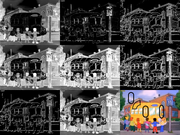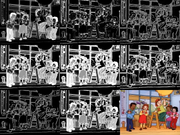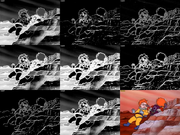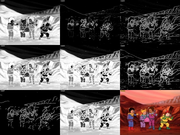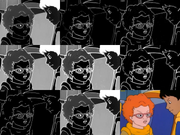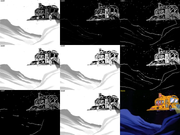masq8 is somewhat decent. But I still miss some edges, from the bus on frame 44 for example. The one I drawed on. There's noise between the edges of the bus windows and around the flagpole, so when I try to avoid those I have trouble gettind all the edges of the bus or other edges correcly. I tried finedehalo2 dwith default (since I don't know anything about convultions.

Maybe I'm to intense.20th December 2021, 20:58   #12  |  Link
Blankmedia
Registered User

Join Date: Oct 2011
Location: Dans le nord
Posts: 62
Quote:
 Originally Posted by anton_foyWell to simplify it and I may be wrong but wouldn't it be a good idea to filter the lines out first just using coloyuv(autogain=true) and the levels or mt_binarize to threshold down to isolate the black lines as much as possible? Then after this step do the edge detection thingy or what else is needed. I dont know because Im not into cartoons like that but logically this is the approach I would use.
Yes, these are good ideas, but I' not very good playing with levels at least in this case.

It doesn't seem to work because other area of the image seem to fall in the same threshold.

Cartoon edges are tricky, because you need your mask to cover the whole edge vs the 2 edges of the dark line. Else when you filter you can get an empty line.

There migth be something to do with a sequence of logical merge to isolate properly only the edges and real dark areas (like kids air, space, etc).20th December 2021, 21:19   #13  |  Link
Blankmedia
Registered User

Join Date: Oct 2011
Location: Dans le nord
Posts: 62
Quote:
 Originally Posted by DogwayNormally I would run a FastLineDarkenMOD to darken the edges and then run an edge mask. The problem is that as you might know edge masks creates double lines so something smarter has to be done. Luckily I managed to implement a ridge detector into FlatMask(), if you wait a day or so you can find it in my repo.
I'm eager to see it, you make great stuff!

Here are some try I made so far:20th December 2021, 21:36   #14  |  Link
StainlessS
HeartlessS Usurer

Join Date: Dec 2009
Location: Over the rainbow
Posts: 10,540
I have no idea if this is of any use [and dont know much about it], but VcMahon, has below plugin,
its an old plugin, but VC was a gas/oil pipeline guy who I think worked with satellite [maybe RADAR/SONAR etc] and other imagery
to try segment/partition and extract detail from them, those techniques might be applicable to cartoon stuff.
Anyways, might be worth a gander at least.

Image enhancement using watershed technique:- https://forum.doom9.org/showthread.php?t=117710

Quote:
 Originally Posted by vcmohanI have recently authored two plugins using Segmentation by watershed algorithm. SegmentedAmp plugin smooths image within the basin boundaries and also accentuates the boundaries. By preprocessing the image to reduce over_segmentation, image can be enhanced. ReTouch plugin in addition to segmentation uses flood fill technique to stretch image values within the basin boundaries or shifts or replaces by the lowest or highest value within each basin. I have used an image which appeared in one of the threads and didee wove a magic spell around it. http://forum.doom9.org/showthread.php?t=115181 The result from using segmentedAmp are :Top row are inputs with various preprocessing plugins. Result with ReTouch is :On right is processed using retouch and other plugins. Only spatial processing has been done. More details are at my plugin page.
EDIT: The images have disappeared from the thread.

EDIT: VC, still visits the forum quite regularly.
__________________
I sometimes post sober.
StainlessS@MediaFire ::: AND/OR ::: StainlessS@SendSpace

"Some infinities are bigger than other infinities", but how many of them are infinitely bigger ???

Last edited by StainlessS; 20th December 2021 at 21:52.21st December 2021, 03:52   #15  |  Link
Blankmedia
Registered User

Join Date: Oct 2011
Location: Dans le nord
Posts: 62
Quote:
 Originally Posted by StainlessSI have no idea if this is of any use [and dont know much about it], but VcMahon, has below plugin, its an old plugin, but VC was a gas/oil pipeline guy who I think worked with satellite [maybe RADAR/SONAR etc] and other imagery to try segment/partition and extract detail from them, those techniques might be applicable to cartoon stuff. Anyways, might be worth a gander at least. Image enhancement using watershed technique:- https://forum.doom9.org/showthread.php?t=117710 EDIT: The images have disappeared from the thread. Sonar Imagery:- https://www.google.co.uk/search?q=so...client=gws-wiz Radar Imagery:- https://www.google.co.uk/search?q=ra...client=gws-wiz satellite imagery:- https://www.google.co.uk/search?q=sa...client=gws-wiz EDIT: VC, still visits the forum quite regularly.
Neat, I'll look up that thread21st December 2021, 07:10 #16  |  Link Blankmedia Registered User   Join Date: Oct 2011 Location: Dans le nord Posts: 62 Hot damn, I have to go to sleep, I'm not sure what did the trick. It's not final but some undetectable edges are now visible and the mask is manageable. But before I used V. C. Mohan plugin now called ManyPlus, I remembered NonLinUSM. It sure did help by enhancing the edges a bit. I also stack a couple of low fastlinedarkenmod ans motion compensated sharpening. I also got better with levels. I'm not sure it's worth it, but so far it's not that bad fps wise, and there's clearly to much stuff here. I might not need that TemporalDegrain2 call. Code: `FPS (min | max | average): 1.796 | 4.623 | 2.053` Code: ```FFMS2(chemin) RoboCrop(LeftAdd=2, TopAdd=2, RightAdd=2, BotAdd =2, align=True) ColorYUV(autogain=True) source=last dn = frfun7(lambda=1.1, T=6.0, Tuv=2.0, p=1).FastLineDarkenMOD4(strength=255, prot=40, luma_cap=250, threshold=4, thinning=5).FastLineDarkenMOD4(strength=255, prot=40, luma_cap=250, threshold=4, thinning=5) dn = dn.FastLineDarkenMOD4(strength=255, prot=40, luma_cap=250, threshold=4, thinning=5).FastLineDarkenMOD4(strength=255, prot=40, luma_cap=250, threshold=4, thinning=5).TemporalDegrain2(degrainTR=2, grainLevel=2, postFFT=3, postTR=1, postSigma=1.0, postDither=-1, postMix=0, extraSharp=False, fftThreads=1, debug=false) #~ gb = ex_GaussianBlur(source, sigma=1.0, pad=true, mblur=false).FastLineDarkenMOD4(strength=255, prot=40, luma_cap=250, threshold=4, thinning=5) gb = dn sharp0 = gb.NonlinUSM(z=3, pow=4.0, str=1.5, rad=9.0).FastLineDarkenMOD4(strength=255, prot=0, luma_cap=255, threshold=4, thinning=5).mergechroma(source) sup1 = gb.MSuper(pel=2,sharp=2) sup2 = sharp0.MSuper(pel=2,sharp=2,levels=1) bv1 = sup1.MAnalyse(isb=true, delta=1) fv1 = sup1.MAnalyse(isb=false,delta=1) bv2 = sup1.MAnalyse(isb=true, delta=2) fv2 = sup1.MAnalyse(isb=false,delta=2) gb = gb.MDegrain2(sup2,bv1,fv1,bv2,fv2) gb = ex_blur(1.5,mode="gaussian",UV=3).FastLineDarkenMOD4(strength=255, prot=40, luma_cap=250, threshold=4, thinning=5).NonlinUSM(pow=4) sh2=manyPlus_SegAmp(source, useclip = false, sh =-6,sm =6) sh1=manyPlus_SegAmp(dn, useclip = false, sh = -2, sm = 10 , smu = 10, smv = 10) sh0 =manyPlus_SegAmp(gb.drk(), useclip = False, sh = -1, sm = 3, smu = 5, smv = 5,sclip=sh1 ) #~ return sh1 s1 = stackhorizontal(source,gb,dn) s2 = stackhorizontal(sh2,sh0, sh1) #~ return stackvertical(s1,s2) #~ manyPlus_SegAmp(dn, useclip = false, sh = 0, sm = 2, smu=2,smv=2,c4=True) #~ frfun7(lambda=1.1, T=6.0, Tuv=2.0, p=1) #~ lumamask(b=0,w=250,UV=128).Turnleft().maa2().TurnRight() #~ Finedehalo2().HaloBuster(h=16, thr=1, elast=3)#.hqdn3d(ls=4.0, cs=3.0, lt=6.0, ct=4.5, restart=7, u=2, v=2)#.hqderingmod(mthr =140,minp = 1, mrad=4, nrmode = 3, show =False) #~ Spline64Resize(1440,1080) source=sh0 #~ TemporalDegrain2(degrainTR=2, grainLevel=2, postFFT=3, postTR=1, postSigma=1.0, postDither=-1, postMix=0, extraSharp=False, fftThreads=1, debug=false) w = Width() h = Height() source.Spline64Resize(w*3, h*3, src_left=0.0, src_top=0.0, src_width=0.0, src_height=0.0) /* masq1 = ex_edge("FDoG", 30, 80, invert=False, UV=128) #30-80 masq2 = ex_edge("cartoon", 0, 5, invert=False, UV=128) #30-80 masq2 = masq2.RemoveGrain(26) ex_logic(masq1, masq2, "or") */ #~ FineDehalo2().HQderingmod(mrad=3) ColorYUV(autowhite=False, autogain=True) Levels(20, 0.50, 200, 0, 255, coring=true) sourcelevel = last blanc = BlankClip(last, color=\$000000).Extracty() masq1 = GibbsNoiseBlock(mode_RG=26).neo_minideen(3).lumamask(b=20,w=200,UV=128).Turnleft().maa2().TurnRight().Extracty().mt_expand_multi(mode="ellipse",sw=2,sh=2).mt_inpand_multi(mode="ellipse",sw=2,sh=2).ex_deflate(UV=128).ex_invert(UV=128) #~ masq1 = ex_edge("FDoG", 40, 50, invert=False, UV=128).Extracty() #30-80 masq2 = Flatmask(rad=1, hyst=1).mt_inpand_multi(mode="ellipse",sw=1,sh=1).ex_inflate(UV=128) #.mt_expand_multi(mode="ellipse",sw=3,sh=3).mt_inpand_multi(mode="ellipse",sw=4,sh=4) masq3 = ex_edge("cartoon", 1, 3, invert=False, UV=128).Removegrain(4).neo_minideen(3).Extracty().ex_inflate(UV=128) #30-80 masq4 = ex_logic(masq1, masq2, "or").Removegrain(1).Extracty().ex_inflate(UV=128) masq5 = ex_logic(masq3, masq4, "or").Removegrain(4).ex_inflate(UV=128)#.ex_invert(UV=128) masq6= ex_logic(masq3, masq2, "or").Removegrain(4).Extracty().maa2().ex_inflate(UV=128) #~ masq6= ex_logic(masq6, masq5, "or").Removegrain(1).Extracty().mt_inpand_multi(mode="ellipse",sw=2,sh=2).ex_binarize(20,UV=128).ex_blur(radius=2, radiusV=2, UV=128).ex_inflate(UV=128) noisymasq = mt_edge(thy1=2.,thy2=2.,mode="cartoon") cleanmasq = mt_edge(thy1=6,thy2=6,mode="cartoon") masq7 = mt_hysteresis(cleanmasq,noisymasq).Extracty().Removegrain(4).mt_inflate().ex_blur(radius=2, radiusV=2, UV=128).maa2().ex_inpand(1).ex_binarize() masq8 = ex_logic(masq4, masq7, "or").Removegrain(26).Extracty().ex_inflate(UV=128).mt_inpand_multi(mode="ellipse",sw=2,sh=2) masq9 = mt_edge(thy1=20,thy2=20,mode="cartoon",chroma="-128").Extracty().ex_inpand(1) masq10 = mt_hysteresis(masq9,masq7).Extracty().Removegrain(4).maa2() #~ return StackHorizontal(masq10, masq9, masq7) ligne1 = StackHorizontal(masq1.Spline64Resize(720,540).Subtitle("masq1").Converttoyv12(),masq2.Spline64Resize(720,540).Subtitle("masq2").Converttoyv12(), masq3.Spline64Resize(720,540).Subtitle("masq3").Converttoyv12()) ligne2 = StackHorizontal(masq4.Spline64Resize(720,540).Subtitle("masq4").Converttoyv12(),masq5.Spline64Resize(720,540).Subtitle("masq5").Converttoyv12(),masq6.Spline64Resize(720,540).Subtitle("masq6").Converttoyv12()) ligne3 = StackHorizontal(masq7.Spline64Resize(720,540).Subtitle("masq7").Converttoyv12(),masq8.Spline64Resize(720,540).Subtitle("masq8").Converttoyv12(), Source.Spline64Resize(720,540).Subtitle("Source").ShowFrameNumber( x= 640, y = 24, text_color=\$ff0000).Converttoyv12()) ligne4 = StackHorizontal(masq9.Spline64Resize(720,540).Subtitle("masq9").Converttoyv12(),masq10.Spline64Resize(720,540).Subtitle("masq10").Converttoyv12(), sourcelevel.Spline64Resize(720,540).Subtitle("sourcelevel").ShowFrameNumber( x= 640, y = 24, text_color=\$ff0000).Converttoyv12()) stack = Stackvertical(ligne1, ligne2, ligne3, ligne4) return stack``` It's on the right path.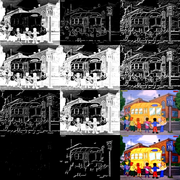21st December 2021, 10:56 #17  |  Link Dogway Registered User   Join Date: Nov 2009 Posts: 2,095 I finished the refactor of FlatMask() with a proper ridge descriptor. I didn't follow the equations to the letter but got the grasp of it and it works. I focused on the double line topic of the original post. To make the faint edge of the bus visible, in the edge mask you have to really pump up the values and hence will catch a lot of false positives because actually it isn't a line anymore but a slight shade.The source is dirty (dot crawl, halos...) so I added some prefiltering before flatmask. Code: ```a=last # Prefilter (Custom for this source) ex_boxblur(0,1,mode="mean") turnright() ex_median("verticalS") turnleft() ex_median("IQM") # Valley Mask FlatMask(1) # Add regular edge mask a=a.ex_edge("qprewitt") ex_lutxy(a,"x dup * 0.1 * y + 0.5 *") # Clean up ex_smooth(2,sharp=true)``` __________________ i7-4790K@Stock::GTX 1070] AviSynth+ filters and mods on GitHub + Discussion thread Last edited by Dogway; 21st December 2021 at 11:14.21st December 2021, 16:21   #18  |  Link
SaurusX
Registered User

Join Date: Feb 2017
Posts: 113
Quote:
 Originally Posted by DogwayI finished the refactor of FlatMask() with a proper ridge descriptor. I didn't follow the equations to the letter but got the grasp of it and it works. I focused on the double line topic of the original post. To make the faint edge of the bus visible, in the edge mask you have to really pump up the values and hence will catch a lot of false positives because actually it isn't a line anymore but a slight shade.The source is dirty (dot crawl, halos...) so I added some prefiltering before flatmask. Code: ```a=last # Prefilter (Custom for this source) ex_boxblur(0,1,mode="mean") turnright() ex_median("verticalS") turnleft() ex_median("IQM") # Valley Mask FlatMask(1) # Add regular edge mask a=a.ex_edge("qprewitt") ex_lutxy(a,"x dup * 0.1 * y + 0.5 *") # Clean up ex_smooth(2,sharp=true)```
Very impressive!22nd December 2021, 04:34 #19  |  Link Blankmedia Registered User   Join Date: Oct 2011 Location: Dans le nord Posts: 62 Thanks Dogway I used your code and it's amazing! Also I added a bit of a luma mask (thanks again) and a debanding call after your cleanup. I made 2 Hqderingmod call to reduce some parallel lines, but I'm not sure yet if I' gonna keep it. It seems to give consistent result : Code: ```src = FFMS2() src.HQderingmod(mrad=2,minp = 2 , nrmode =0, sharp = 0, show=False).HQderingmod(mrad=2,minp = 2 , nrmode =3, sharp = 0, show=False) a = last ex_boxblur(0,1,mode="mean") turnright() ex_median("verticalS") turnleft() ex_median("IQM") neo_f3kdb(5,preset="very high",grainy=0, grainc=0, blur_first=true, sample_mode=4) # Add lumamask to get dark haris and shoes and stuff, also limit texture degradation in dark area lumen = lumamask(b=00,w=50, invert=True,uv=128).Santiag().ex_inflate(1,uv=128).Converttoy() lumen = lumen.Levels(40,0.7,240,0,255) # Valley Mask dana = FlatMask(1) # Add regular edge mask a=a.ex_edge("qprewitt").Converttoy() emask = dana.ex_lutxy(a,"x dup * 0.1 * y + 0.5 *") #~ emask = ex_logic(emask, lumen, "or") ## create artefact emask = emask.ex_lutxy(lumen,"y x > y x ?") # Clean up emask.ex_smooth(2,sharp=true)``` This is my whole script so far, but it needs tuning a bit. I went way over board with a lot of things. Code: ```FFMS2(chemin) RoboCrop(LeftAdd=2, TopAdd=2, RightAdd=2, BotAdd =2, align=True) fmtc_bitdepth(bits=32) fmtc_resample(css="444", fulls=False, fulld=True) fmtc_matrix(mats="601", matd="709", fulls=True, fulld=True) fmtc_matrix(mat="709", fulls=True, fulld=False, col_fam="YUV") fmtc_resample (css="420") src16 = fmtc_bitdepth(bits=16, dmode=8) fmtc_bitdepth(bits=8, dmode=8) src = last HQderingmod(mrad=2,minp = 2 , nrmode =0, sharp = 0, show=False).HQderingmod(mrad=2,minp = 2 , nrmode =3, sharp = 0, show=False) ex_boxblur(0,1,mode="mean") turnright() ex_median("verticalS") turnleft() ex_median("IQM") neo_f3kdb(5,preset="very high",grainy=0, grainc=0, blur_first=true, sample_mode=4) # Add lumamask to get dark haris and shoes and stuff, also limit texture degradation in dark area lumen = lumamask(b=00,w=50, invert=True,uv=128).Santiag().ex_inflate(1,uv=128).Converttoy() lumen = lumen.Levels(40,0.7,240,0,255) # Valley Mask dana = FlatMask(1) # Add regular edge mask a=src.ex_edge("qprewitt").Converttoy() emask = dana.ex_lutxy(a,"x dup * 0.1 * y + 0.5 *") emask = emask.ex_lutxy(lumen,"y x > y x ?") # Clean up emask = emask.ex_smooth(2,sharp=true) emask16 = emask.fmtc_bitdepth(bits=16) src16= src16.GibbsNoiseBlock(mode_RG=26) sharp0 = src16.NonlinUSM(z=3, pow=1.1, str=0.25, rad=9).mergechroma(src16) sup1 = src16.MSuper(pel=2,sharp=2) sup2 = sharp0.MSuper(pel=2,sharp=2,levels=1) bv1 = sup1.MAnalyse(isb=true, delta=1) fv1 = sup1.MAnalyse(isb=false,delta=1) bv2 = sup1.MAnalyse(isb=true, delta=2) fv2 = sup1.MAnalyse(isb=false,delta=2) sharpy = src16.MDegrain2(sup2,bv1,fv1,bv2,fv2) denoised = src16.GibbsNoiseBlock(mode_RG=26).minideen(radius=1).Halobuster(h=12) denoised = denoised.TemporalDegrain2(degrainTR=1, grainLevel=1, postFFT=0, postTR=1, postSigma=1.0, postDither=-1, postMix=0, extraSharp=True, fftThreads=1, debug=false) ex_merge(denoised, sharpy, emask16,true).maa2() DFMDeRainbow(maskthresh=12) EDI_RPow2(4,CShift="Spline64Resize",FWidth=960,FHeight=720, nsize=0, nns=3, qual=2, etype=1, taps=4) emask16 = emask16.spline64resize(960,720) source = last sharp0 = source.mt_adddiff(mt_makediff(source,source.removegrain(4)),U=2,V=2) # "median sharpen" (won't create halos on its own, IF the source is halo-free) sup1 = source.MSuper(pel=2,sharp=2) sup2 = sharp0.MSuper(pel=2,sharp=2,levels=1) bv1 = sup1.MAnalyse(isb=true, delta=1) fv1 = sup1.MAnalyse(isb=false,delta=1) bc1 = source.MCompensate(sup2,bv1,thSAD=500) # using the super clip from the median-sharpener, to provide fc1 = source.MCompensate(sup2,fv1,thSAD=500) # more headroom for the limiting process max = mt_logic(bc1,fc1,"max").mt_logic(source,"max") min = mt_logic(bc1,fc1,"min").mt_logic(source,"min") sharp1 = source.LSFmod(preset="slow", strength=200, smode=5, edgemode=1).CAS(0.5).mergechroma(last) # chroma sharpen usually is unadvantageous sharpy2 = sharp1.mt_clamp(max,min,0,0,U=2,V=2).FastLineDarkenMOD4(strength=75, prot=60, luma_cap=190, threshold=4, thinning=1).FastLineDarkenMOD4(strength=75, prot=60, luma_cap=190, threshold=4, thinning=1) #~ shapy2 ex_merge(source, sharpy2, emask16,true).maa2() emask16 = emask16.ex_inpand(1,"plus",thres=128) deh = source.FineDehalo(rx=2.5, ry=2.5, thmi=80, thma=128, thlimi=50, thlima=100, darkstr=0.3, brightstr=1.0, showmask=0, contra=0.3, excl=true) ex_merge(deh, last, emask16,true) w=656 h=480 fr=44 a1 = src16.Trim(fr, fr).Spline64Resize(w,h).Subtitle("Source frame" + String(fr)) a2 = emask16.Trim(fr, fr).Spline64Resize(w,h).Subtitle("Mask frame" + String(fr)).ConvertToYUV420() a3 = last.Trim(fr, fr).Spline64Resize(w,h).Subtitle("Result frame" + String(fr)) ligne1 = Stackhorizontal(a1, a3, a2) fr=77 a1 = src16.Trim(fr, fr).Spline64Resize(w,h).Subtitle("Source frame" + String(fr)) a2 = emask16.Trim(fr, fr).Spline64Resize(w,h).Subtitle("Mask frame" + String(fr)).ConvertToYUV420() a3 = last.Trim(fr, fr).Spline64Resize(w,h).Subtitle("Result frame" + String(fr)) ligne2 = Stackhorizontal(a1, a3, a2) fr=5000 a1 = src16.Trim(fr, fr).Spline64Resize(w,h).Subtitle("Source frame" + String(fr)) a2 = emask16.Trim(fr, fr).Spline64Resize(w,h).Subtitle("Mask frame" + String(fr)).ConvertToYUV420() a3 = last.Trim(fr, fr).Spline64Resize(w,h).Subtitle("Result frame" + String(fr)) ligne3 = Stackhorizontal(a1, a3, a2) fr=10000 a1 = src16.Trim(fr, fr).Spline64Resize(w,h).Subtitle("Source frame" + String(fr)) a2 = emask16.Trim(fr, fr).Spline64Resize(w,h).Subtitle("Mask frame" + String(fr)).ConvertToYUV420() a3 = last.Trim(fr, fr).Spline64Resize(w,h).Subtitle("Result frame" + String(fr)) ligne4 = Stackhorizontal(a1, a3, a2) fr=15000 a1 = src16.Trim(fr, fr).Spline64Resize(w,h).Subtitle("Source frame" + String(fr)) a2 = emask16.Trim(fr, fr).Spline64Resize(w,h).Subtitle("Mask frame" + String(fr)).ConvertToYUV420() a3 = last.Trim(fr, fr).Spline64Resize(w,h).Subtitle("Result frame" + String(fr)) ligne5 = Stackhorizontal(a1, a3, a2) fr=20000 a1 = src16.Trim(fr, fr).Spline64Resize(w,h).Subtitle("Source frame" + String(fr)) a2 = emask16.Trim(fr, fr).Spline64Resize(w,h).Subtitle("Mask frame" + String(fr)).ConvertToYUV420() a3 = last.Trim(fr, fr).Spline64Resize(w,h).Subtitle("Result frame" + String(fr)) ligne6 = Stackhorizontal(a1, a3, a2) fr=25000 a1 = src16.Trim(fr, fr).Spline64Resize(w,h).Subtitle("Source frame" + String(fr)) a2 = emask16.Trim(fr, fr).Spline64Resize(w,h).Subtitle("Mask frame" + String(fr)).ConvertToYUV420() a3 = last.Trim(fr, fr).Spline64Resize(w,h).Subtitle("Result frame" + String(fr)) ligne7 = Stackhorizontal(a1, a3, a2) fr=30000 a1 = src16.Trim(fr, fr).Spline64Resize(w,h).Subtitle("Source frame" + String(fr)) a2 = emask16.Trim(fr, fr).Spline64Resize(w,h).Subtitle("Mask frame" + String(fr)).ConvertToYUV420() a3 = last.Trim(fr, fr).Spline64Resize(w,h).Subtitle("Result frame" + String(fr)) ligne8 = Stackhorizontal(a1, a3, a2) stack = Stackvertical(ligne1, ligne2, ligne3, ligne4, ligne5, ligne6, ligne7, ligne8) return stack #~ ConvertToStacked().Dither_out() Prefetch(threads=1)```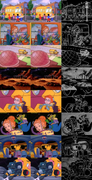Zoom in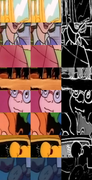Thanks to everyone again. Last edited by Blankmedia; 22nd December 2021 at 04:53.22nd December 2021, 07:13 #20  |  Link Blankmedia Registered User   Join Date: Oct 2011 Location: Dans le nord Posts: 62 Im happy as a phoque right now, I'fhink tomorrow I'll reverse de projec to protect the eyes and the stars. I'm encoding a little sample for you all. I should be available now but I must sleep. Envoyé de mon Pixel 6 en utilisant TapatalkTags masksThread Tools Search this ThreadShow Printable VersionEmail this Page Search this Thread: Advanced Search Display ModesLinear ModeSwitch to Hybrid ModeSwitch to Threaded ModePosting Rules You may not post new threads You may not post replies You may not post attachments You may not edit your posts BB code is On Smilies are On [IMG] code is On HTML code is Off Forum Rules
 Forum Jump User Control Panel Private Messages Subscriptions Who's Online Search Forums Forums Home Announcements and Chat     General Discussion     News     Forum / Site Suggestions & Help General     Decrypting     Newbies     DVD2AVI / DGIndex     Audio encoding     Subtitles     Linux, Mac OS X, & Co Capturing and Editing Video     Avisynth Usage     Avisynth Development     VapourSynth     Capturing Video     DV     HDTV / DVB / TiVo     NLE - Non Linear Editing     VirtualDub, VDubMod & AviDemux     New and alternative a/v containers Video Encoding     (Auto) Gordian Knot     MPEG-4 ASP     MPEG-4 Encoder GUIs     MPEG-4 AVC / H.264     High Efficiency Video Coding (HEVC)     New and alternative video codecs     MPEG-2 Encoding     VP9 and AV1 (HD) DVD, Blu-ray & (S)VCD     One click suites for DVD backup and DVD creation     DVD & BD Rebuilder     (HD) DVD & Blu-ray authoring     Advanced authoring     IFO/VOB Editors     DVD burning Hardware & Software     Software players     Hardware players     PC Hard & Software Programming and Hacking     Development     Translations

All times are GMT +1. The time now is 14:02.

 Doom9.org - Archive - Top